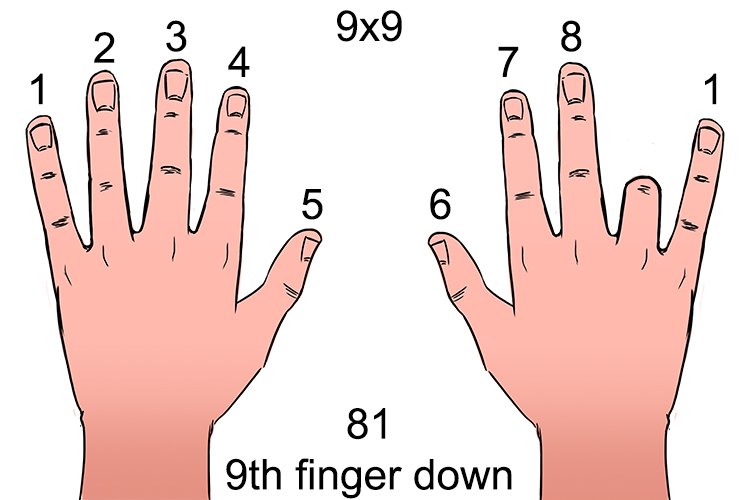# 9 Times table

Nine times table
Multiplier less one = 1st N^o
9 minus 1st N^o = 2nd N^o

Take one from the other multiplier and this gives the first number. Take this first number away from nine and you have your second number. The first number and second number always add up to nine.

i.e.

2times9   :    (2-1)=1   and   9 - 1=8               2times9= 18

3times9   :    (3-1)=2    and    9 - 2=7               3times9= 27

4times9   :    (4-1)=3    and   9 - 3=6               4times9= 36

5times9   :    (5-1)=4   and   9 - 4=5               5times9= 45

6times9   :    (6-1)=5   and   9 - 5=4               6times9= 54

7times9   :    (7-1)=6   and   9 - 6=3               7times9= 63

8times9   :    (8-1)=7   and   9 - 7=2               8times9= 72

9times9   :    (9-1)=8   and   9 - 8=1               9times9= 81

10times9 : (10-1)=9   and   9 - 9=0               10times9= 90

11times9 : 11times9=99 (multiplier 9 is repeated - see 11 times tables)

12times9 : 10times9+9+9=90+9+9=108 (see 12 times tables)

NOTE:

1 and 8, 2 and 7, 3 and 6, 4 and 5, 5 and 4, 6 and 3, 7 and 2, 8 and 1, 9 and 0 all add up to nine

e.g   :    2times9  :    2 - 1=1  and   9 - 1=8   :    2times9=18

## 9 Times fingers

Put your hands out in front of you. If your fingers are numbered from one to ten, drop the finger number you're multiplying by 9 and read your hand.

(See how many fingers before and after the bent finger)

Here’s a visual way of doing the 9 times table.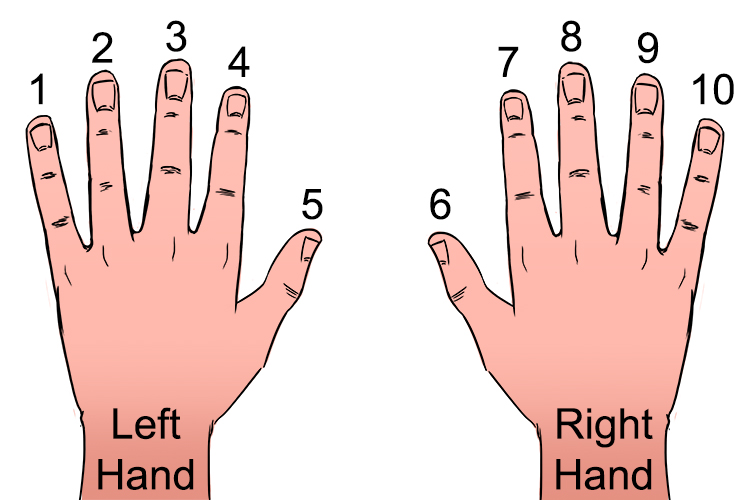Examples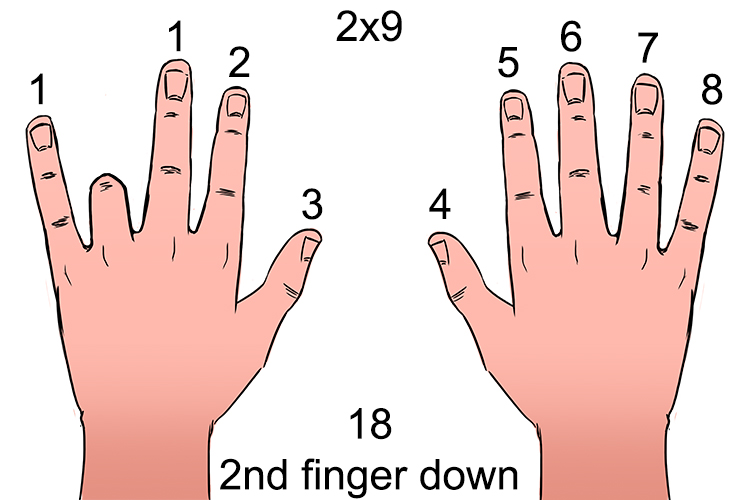Follow a similar procedure for each of the following:

NOTE:

1 finger before the bent finger

8 fingers after the bent finger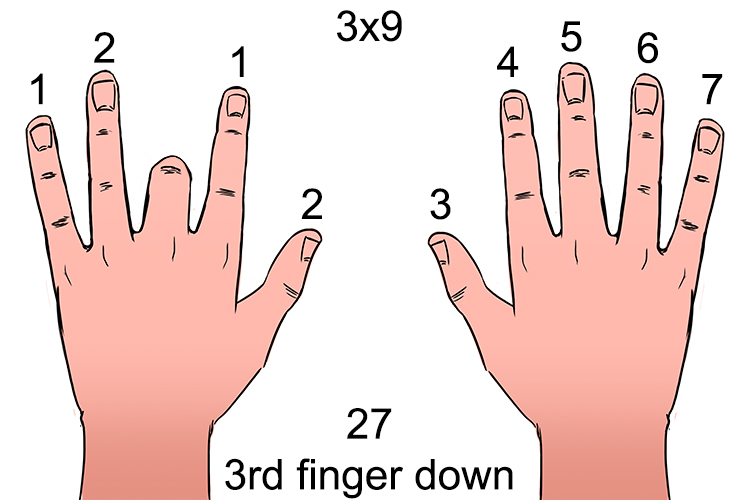NOTE:

2 finger before the bent finger

7 fingers after the bent finger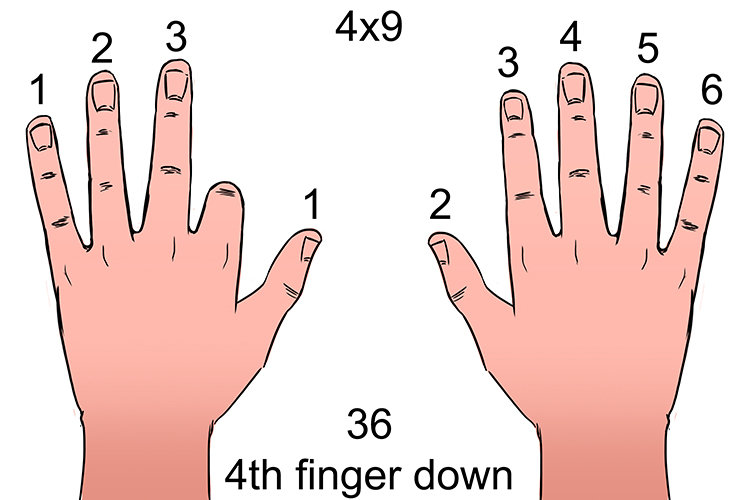NOTE:

3 finger before the bent finger

6 fingers after the bent finger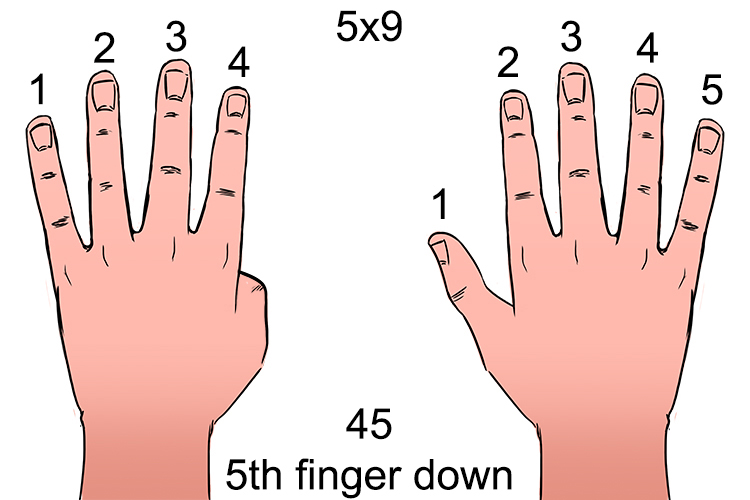NOTE:

4 finger before the bent finger

5 fingers after the bent finger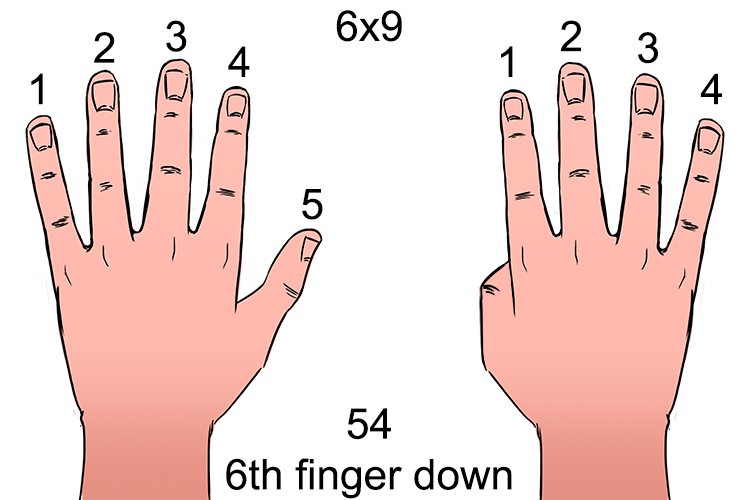NOTE:

5 finger before the bent finger

4 fingers after the bent finger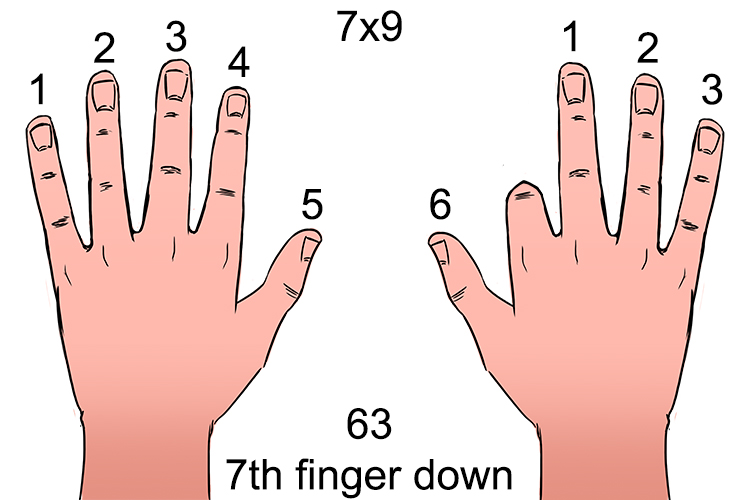NOTE:

6 finger before the bent finger

3 fingers after the bent finger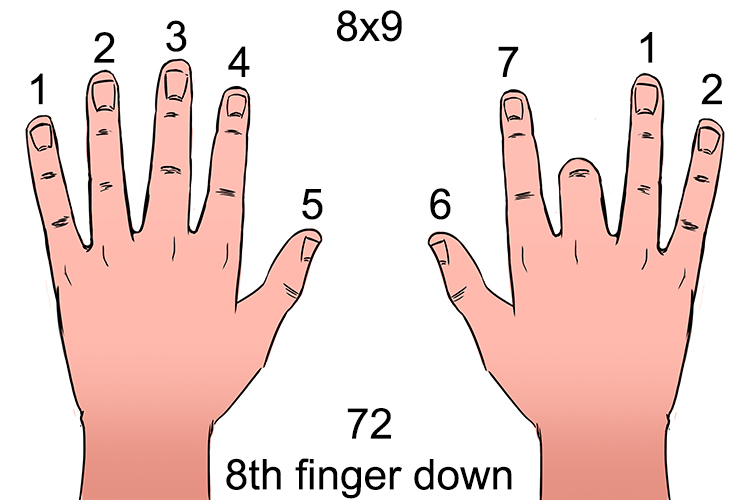NOTE:

7 finger before the bent finger

2 fingers after the bent finger